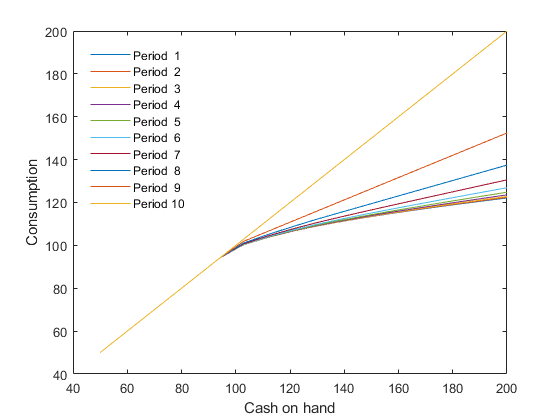# CS2 Finite horizon consumption/saving model with borrowing constraint

This is the same problem as CS1, except that it has a finite horizon.

## Create the model object

model = recsmodel('cs1.yaml',...
struct('Mu',100,'Sigma',10^2,'order',5));

Deterministic steady state (equal to first guess)
State variables:
X
___

100

Response variables:
C
___

100

Expectations variables:
E
______

0.0001



## Define approximation space

[interp,s] = recsinterpinit(20,model.sss/2,model.sss*2);


## Solve for rational expectations with finite horizon

First-guess: Consumption equal to cash on hand

x          = s;


In the last period, $T=10$, the consumer consumes all her cash on hand, $C_T=X_T$:

T          = 10;
xT         = s;
[interp,X] = recsSolveREEFiniteHorizon(interp,model,s,x,xT,T);


## Plot the decision rules

figure
plot(s,squeeze(X))
legend([repmat('Period ',10,1),num2str((1:10)')])
legend('Location','NorthWest')
legend('boxoff')
xlabel('Cash on hand')
ylabel('Consumption')